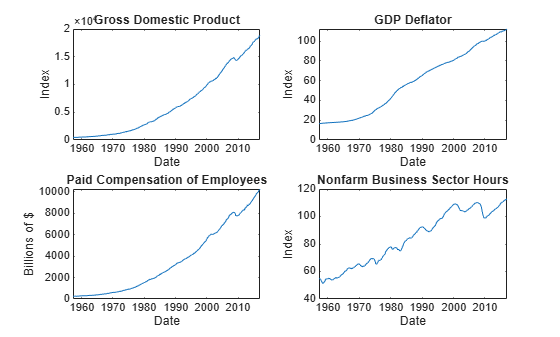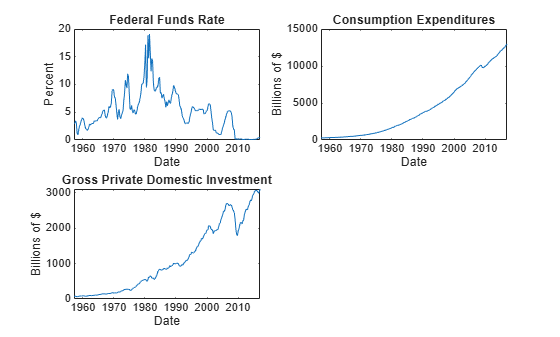varm

Convert vector error-correction (VEC) model to vector autoregression (VAR) model

Description

example

VARMdl = varm(Mdl) converts the VEC(p – 1) model Mdl to its equivalent VAR(p) model representation VARMdl.

Examples

collapse all

Consider a VEC model for the following seven macroeconomic series.

• Gross domestic product (GDP)

• GDP implicit price deflator

• Paid compensation of employees

• Nonfarm business sector hours of all persons

• Effective federal funds rate

• Personal consumption expenditures

• Gross private domestic investment

Suppose that a cointegrating rank of 4 and one short-run term are appropriate, that is, consider a VEC(1) model.

For more information on the data set and variables, enter Description at the command line.

Determine whether the data needs to be preprocessed by plotting the series on separate plots.

figure;
subplot(2,2,1)
plot(FRED.Time,FRED.GDP);
title('Gross Domestic Product');
ylabel('Index');
xlabel('Date');
subplot(2,2,2)
plot(FRED.Time,FRED.GDPDEF);
title('GDP Deflator');
ylabel('Index');
xlabel('Date');
subplot(2,2,3)
plot(FRED.Time,FRED.COE);
title('Paid Compensation of Employees');
ylabel('Billions of \$');
xlabel('Date');
subplot(2,2,4)
plot(FRED.Time,FRED.HOANBS);
ylabel('Index');
xlabel('Date');figure;
subplot(2,2,1)
plot(FRED.Time,FRED.FEDFUNDS);
title('Federal Funds Rate');
ylabel('Percent');
xlabel('Date');
subplot(2,2,2)
plot(FRED.Time,FRED.PCEC);
title('Consumption Expenditures');
ylabel('Billions of \$');
xlabel('Date');
subplot(2,2,3)
plot(FRED.Time,FRED.GPDI);
title('Gross Private Domestic Investment');
ylabel('Billions of \$');
xlabel('Date');Stabilize all series, except the federal funds rate, by applying the log transform. Scale the resulting series by 100 so that all series are on the same scale.

FRED.GDP = 100*log(FRED.GDP);
FRED.GDPDEF = 100*log(FRED.GDPDEF);
FRED.COE = 100*log(FRED.COE);
FRED.HOANBS = 100*log(FRED.HOANBS);
FRED.PCEC = 100*log(FRED.PCEC);
FRED.GPDI = 100*log(FRED.GPDI);

Create a VEC(1) model using the shorthand syntax. Specify the variable names.

Mdl = vecm(7,4,1);
Mdl.SeriesNames = FRED.Properties.VariableNames
Mdl =
vecm with properties:

Description: "7-Dimensional Rank = 4 VEC(1) Model with Linear Time Trend"
SeriesNames: "GDP"  "GDPDEF"  "COE"  ... and 4 more
NumSeries: 7
Rank: 4
P: 2
Constant: [7×1 vector of NaNs]
Cointegration: [7×4 matrix of NaNs]
Impact: [7×7 matrix of NaNs]
CointegrationConstant: [4×1 vector of NaNs]
CointegrationTrend: [4×1 vector of NaNs]
ShortRun: {7×7 matrix of NaNs} at lag 
Trend: [7×1 vector of NaNs]
Beta: [7×0 matrix]
Covariance: [7×7 matrix of NaNs]

Mdl is a vecm model object. All properties containing NaN values correspond to parameters to be estimated given data.

Estimate the model using the entire data set and the default options.

EstMdl = estimate(Mdl,FRED.Variables)
EstMdl =
vecm with properties:

Description: "7-Dimensional Rank = 4 VEC(1) Model"
SeriesNames: "GDP"  "GDPDEF"  "COE"  ... and 4 more
NumSeries: 7
Rank: 4
P: 2
Constant: [14.1329 8.77841 -7.20359 ... and 4 more]'
Cointegration: [7×4 matrix]
Impact: [7×7 matrix]
CointegrationConstant: [-28.6082 109.555 -77.0912 ... and 1 more]'
CointegrationTrend: [4×1 vector of zeros]
ShortRun: {7×7 matrix} at lag 
Trend: [7×1 vector of zeros]
Beta: [7×0 matrix]
Covariance: [7×7 matrix]

EstMdl is an estimated vecm model object. It is fully specified because all parameters have known values. By default, estimate imposes the constraints of the H1 Johansen VEC model form by removing the cointegrating trend and linear trend terms from the model. Parameter exclusion from estimation is equivalent to imposing equality constraints to zero.

Convert the estimated VEC(1) model to its equivalent VAR(2) model representation.

VARMdl = varm(EstMdl)
VARMdl =
varm with properties:

Description: "AR-Nonstationary 7-Dimensional VAR(2) Model"
SeriesNames: "GDP"  "GDPDEF"  "COE"  ... and 4 more
NumSeries: 7
P: 2
Constant: [14.1329 8.77841 -7.20359 ... and 4 more]'
AR: {7×7 matrices} at lags [1 2]
Trend: [7×1 vector of zeros]
Beta: [7×0 matrix]
Covariance: [7×7 matrix]

VARMdl is a varm model object.

Input Arguments

collapse all

VEC model, specified as a vecm model object created by vecm or estimate. Mdl must be fully specified.

Output Arguments

collapse all

VAR model equivalent, returned as a varm model object.

Algorithms

Consider the m-D VEC(p – 1) model using lag operator notation.

$\left(1-L\right){y}_{t}=c+dt+\Pi {y}_{t-1}+\sum _{j=1}^{p-1}{\Phi }_{j}\left(1-L\right){y}_{t-j}+\beta {x}_{t}+{\epsilon }_{t}.$

• yt is an m-by-1 vector of values corresponding to m response variables at time t, where t = 1,...,T.

• Lyt = yt – 1.

• c is the overall constant.

• d is the overall time trend coefficient.

• Π is an m-by-m impact matrix with a rank of r.

• xt is a k-by-1 vector of values corresponding to k exogenous predictor variables.

• β is an m-by-k matrix of regression coefficients.

• εt is an m-by-1 vector of random Gaussian innovations, each with a mean of 0 and collectively an m-by-m covariance matrix Σ. For ts, εt and εs are independent.

• Φj is an m-by-m matrix of short-run coefficients.

The equivalent VAR(p) model in difference equation notation is

${y}_{t}=c+dt+\sum _{j=1}^{p}{\Gamma }_{j}{y}_{t-j}+\beta {x}_{t}+{\epsilon }_{t}.$

Γj is an m-by-m matrix of autoregressive coefficients.